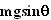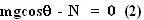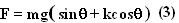Thursday, February 14, 2008

Irodov Problem 1.121Consider a certain section of the hill where the slope is an angle. The mass experiences four forces, i) the force of gravity mg acting vertically downwards, ii) the force of friction along the downward slope of the hill since it opposes the upward motion of the mass, iii) the normal reaction from the surface of the hill and iv) the force F directed along the upward slope of the hill.

Since the mass is being dragged "slowly" there the body experiences no acceleration. So basically the force F constantly exactly compensates and cancels out the force of friction Nk and the component of force of gravity along the slope. In other words,Along the direction normal to the surface there are two forces, i) the normal reaction N and ii) the component of gravity. There is no acceleration along this direction and so we have,From (1) and (2) we have,Now the work done dW at some section of the slope by the force when the mass climbs up a distance ds is given by Fds (since the force always aligns itself along the slope). So we have,From elementary geometry we know that,From (5a) and (5b) we have,